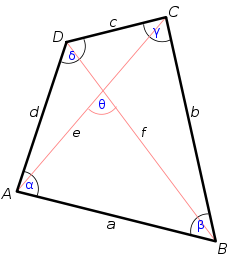# Bretschneider's formulaIn geometry, Bretschneider's formula is the following expression for the area of a general quadrilateral:Here, a, b, c, d are the sides of the quadrilateral, s is the semiperimeter, and α and γ are two opposite angles.

Bretschneider's formula works on any quadrilateral, whether it is cyclic or not.

The German mathematician Carl Anton Bretschneider discovered the formula in 1842. The formula was also derived in the same year by the German mathematician Karl Georg Christian von Staudt.

## Proof

Denote the area of the quadrilateral by K. Then we haveThereforeThe law of cosines implies thatbecause both sides equal the square of the length of the diagonal BD. This can be rewritten asAdding this to the above formula for 4K2 yieldsNote:Following the same steps as in Brahmagupta's formula, this can be written asIntroducing the semiperimeterthe above becomesand Bretschneider's formula follows.

## Related formulas

Bretschneider's formula generalizes Brahmagupta's formula for the area of a cyclic quadrilateral, which in turn generalizes Heron's formula for the area of a triangle.

The trigonometric adjustment in Bretschneider's formula for non-cyclicality of the quadrilateral can be rewritten non-trigonometrically in terms of the sides and the diagonals e and f to give## Notes

1. J. L. Coolidge, "A historically interesting formula for the area of a quadrilateral", American Mathematical Monthly, 46 (1939) 345–347. (JSTOR)
2. E. W. Hobson: A Treatise on Plane Trigonometry. Cambridge University Press, 1918, pp. 204-205